In elementary school arithmetic, we first learn addition and subtraction, using numbers from 1 to 10.

In order to perform addition and subtraction, we need to understand numbers. We have to know the meaning of the numbers from 1 to 10 and what they represent. This will help you calculate the numbers.

First, let’s learn how to count numbers. After that, we will explain how to do addition and subtraction.

## Understanding the Meaning of Numbers

What is the meaning of the number? For example, when you go shopping, you have to calculate numbers. In order to do that, you need to learn the numbers.

So first, let’s learn the numbers from 1 to 10. For example, let’s say there are apples. The number of apples and numbers are as follows.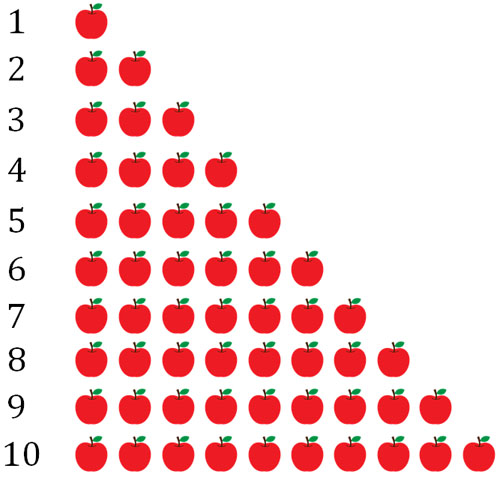Let’s try to match the numbers with the number of apples. For example, how many cars are there in the following?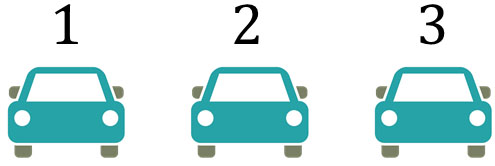If you count the cars in order, you get 1, 2, 3. Therefore, the number of cars is 3.

Also, how many cakes are there in the following?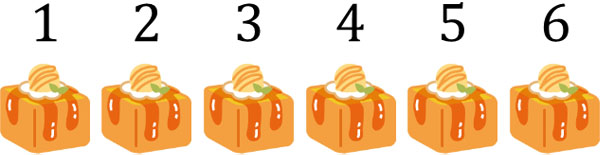In this case, if we count the number of cakes in order, we get 1, 2, 3, 4, 5, 6. Therefore, the number of cakes is 6. In order to do the math, we need to know the meaning of the numbers. So, let’s learn what the numbers 1 to 10 represent.

Next, let’s do an addition. The addition is the process of finding out what happens to numbers when you put things together that are the same. In addition, the symbol $+$ is used. The following is an expression for addition.

• $1+3=4$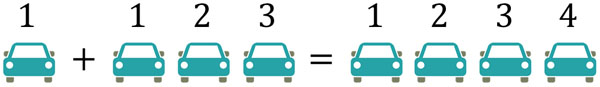There is one car. If we add three more cars, we get a total of four cars.

So, if there are three cakes and four cakes, how many cakes are there in total? Let’s add up 3 cakes and 4 cakes as shown below.

• $3+4=7$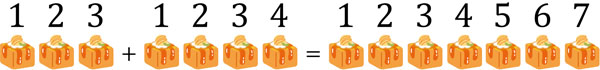In this way, if you add 3 and 4, you get 7. By adding numbers, we can increase the number.

### Adding Numbers from 1 to 10

After learning how to do addition, let’s do addition from 1 to 10. You need to solve a lot of calculation problems like the following.

• $1+4=5$
• $6+1=7$
• $2+3=5$
• $6+4=10$
• $6+3=9$
• $4+5=9$
• $9+1=10$
• $6+2=8$

At first, you can use your fingers to count. There are 10 fingers. So, until you get used to it, use your fingers to do the addition.

For example, let’s do $2+6$. Since it is $2+6$, we use two fingers at first.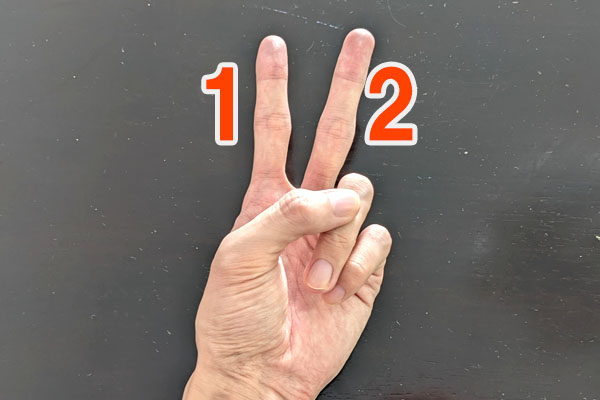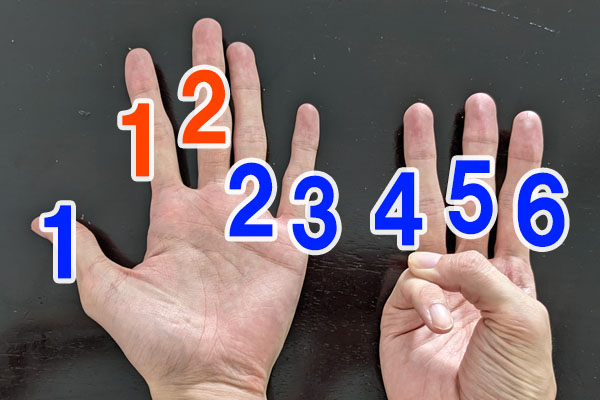Then we know that 8 is the answer.

## What is Subtraction: Calculation by Subtracting Numbers

In math, we learn subtraction as well as addition. In addition, the number increases. In contrast, in subtraction, the number decreases. There are many cases where the number decreases. For example, if you eat apples, the number of apple decreases.

Now let’s learn subtraction. In subtraction, we use the symbol $-$. In subtraction, the equation looks like this.

• $3-2=1$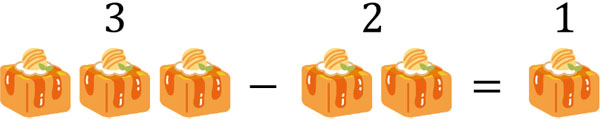There are three cakes. If you eat two cakes, there will be one cake left; out of the three cakes, you have eaten two cakes, so there is only one cake.

Next, let’s consider an apple tree. There are five apples on the tree. If you eat 3 apples, how many apples are left?

• $5-3=2$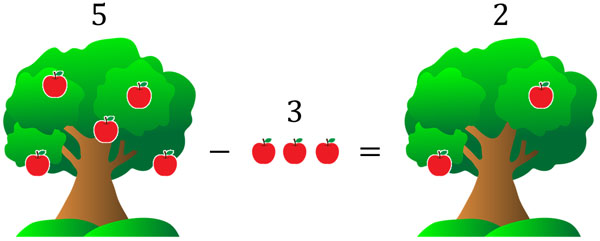If 3 is removed from 5, it becomes 2. Thus, subtraction is the process of reducing a number.

### Subtraction of Numbers from 1 to 10

So, let’s try subtraction using the numbers from 1 to 10. You can solve math problems for subtraction just like you do for addition. It looks like this.

• $4-1=3$
• $6-1=5$
• $3-2=1$
• $6-4=2$
• $10-3=7$
• $8-5=3$
• $9-2=7$
• $6-2=4$

Even if you look at the numbers, you will not be able to do subtraction. So, until you get used to it, use your fingers to calculate what the answer will be.

For example, how do you calculate $7-3$? Since it is $7-3$, let’s make 7 at first.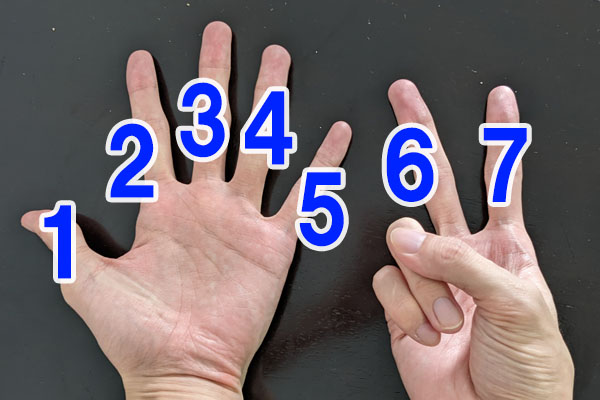The next step is to eliminate three numbers.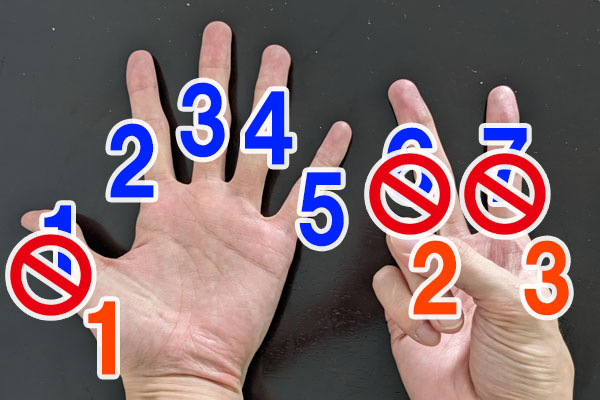As a result, we find that 4 is the answer.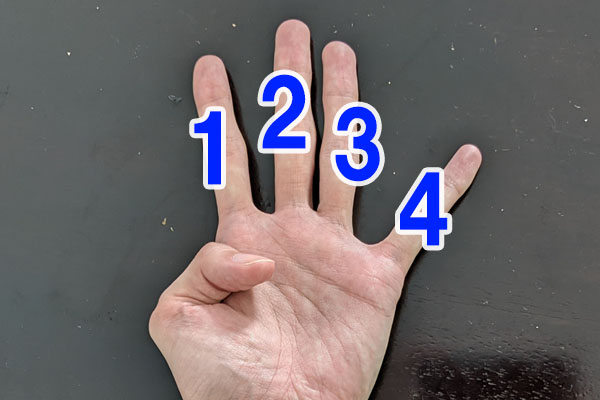In this way, we can calculate subtraction.